### SUMPRODUCT: The Function and Different Formulas

In this lesson you can learn how to use SUMPRODUCT function in Excel.

SUMPRODUCT is the most important (next to SUM) Excel function used in operations research. This function multiplies in the given arrays, and returns the sum of those products.

### Syntax is:

=SUMPRODUCT(array1,[array2],[array3],…)

### Explanation

=SUMPRODUCT (A1:C1,A2:C2) means A1*A2+B1*B2+C1*C2

=SUMPRODUCT(A4:A7,B4:B7) means A4*B4+A5*B5+A6*B6+A7*B7

=SUMPRODUCT(A9:B11,D9:E11) means A9*D9+A10*D10+A11*D11+B9*E9+B10*E10+B11*E11

=SUMPRODUCT(E9:E11,G9:G11) means E9*G9+E10*G10+E11*G11

### Notes:

(array1, [array2], [array3], …) is from 2 to 30 arrays whose elements you multiply and add. Arrays must be in the same dimensions (ranges of cells must have the same number of rows and columns). If it is not, the function SUMPRODUCT returns the #VALUE! error.

### Sum of sales in report

You have sales report. In B column there is count of pieces sold. In C column there are prices. How to calculate sum of sales?Formula here is:

=SUMPRODUCT(B3:B12,C3:C12)

Result is \$223.08.

### Calculating weighted average with Sumproduct function

To calculate weighted average of exam’s result in Excel use below formula:

=SUMPRODUCT(A3:A7,B3:B7)/SUM(B3:B7)As you see points in 5th row are the most important.

### SUMPRODUCT and name ranges

Let’s use the same example. You can name ranges and use them instead of ranges. I named ranges A3:A7 as POINTS and B3:B7 as WAGES.

Now I can change formula

from =SUMPRODUCT(A3:A7,B3:B7)/SUM(B3:B7)

to =SUMPRODUCT(POINTS,WAGES)/SUM(WAGES)More examples of business sumproduct formulas.

### Simple Sumproduct Formula

We are acknowledging the sumproduct of the data that has been layout, and with regards to that, there is need for finding answers to the question. We are using the sumproduct formula to find the answers needed.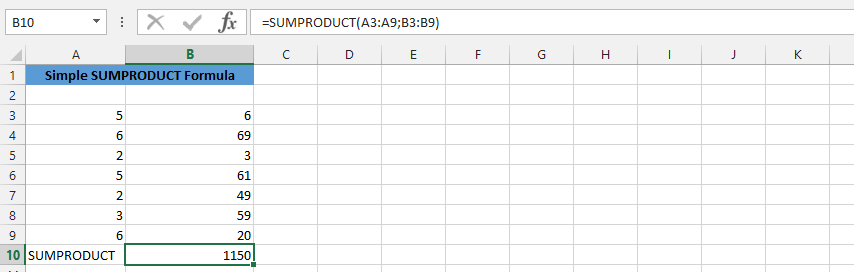=SUMRPODUCT(A3:A9,B3:B9)

### Complex SUMPRODUCT Formula

As we’d know sumproduct would return with the multiplications, and we’d have multiple data that we are trying to find the answers for, which is where we are using the sumproduct formula.=SUMRPODUCT(A3:A9,B3:B9,C3:C9,D3:D9,E3:E9)

### SUMPRODUCT with Flexibilities

There is a percentages that has been layout, and we would like to know the percentage of the sumproduct. This is why we are inputting the percentages that have increased twice, which is why we need to add them.=0.04+0.17+0.29*SUMPRODUCT(A2:I2)

### SUMPRODUCT with Text

The whole data has been labeled with text, and we would need to find answer, which is why we needs to use that label to find the answer we need.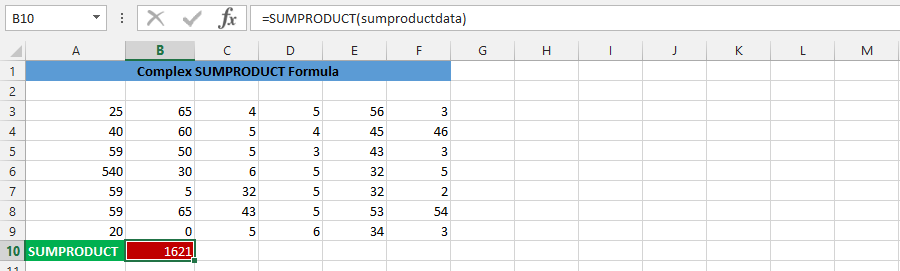=SUMPRODUCT(sumproductdata)

### SUMPRODUCT for Sales

We are using the sumproduct function to find answer for the product, which provides this result.=SUMPRODUCT((A4:A10=5)*(B4:B10=”B”)*(C4:C10))

## SUMPRODUCT and Percentage

We are simultaneously using the sumproduct formulas to get appropriate result with the data we’d have at hand.=SUMPRODUCT(A3:B9,C3:D9,E3:F9)*0.24-0.12+0.06

### Sumproduct in Multiple Cells

We are finding the sumproduct of the data, and use it to get the result in multiple cells. This means, after writing the formula, we’d be pressing F2, followed by ctrl, shift and then press enter on the keyboard.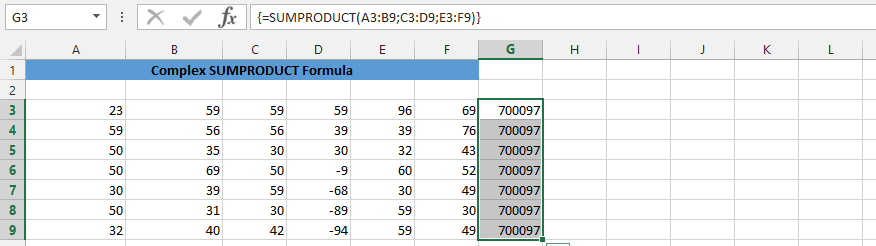{=SUMPRODUCT(A3:B9,C3:D9,E3:F9)}

### Double Sumproduct

There are two competing parties that are trying to get the price, and we are trying to find the winner. This is why we are using two sumproduct formulas to find the winner.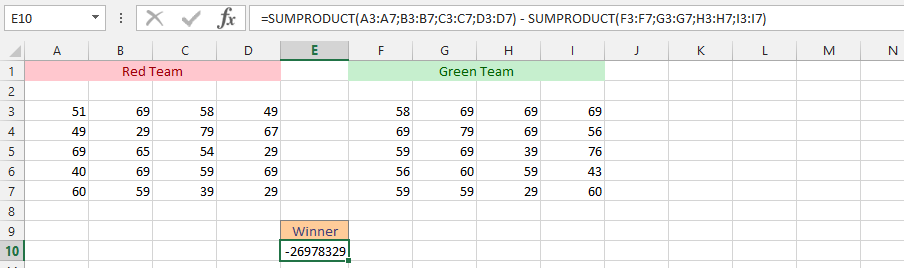=SUMPRODUCT(A3:A7,B3:B7,C3:C7,D3:D7)-SUMPRODUCT(F3:F7,G3:G7,H3:H7,I3:I7)

### IF and SUMPRODUCT

We have the whole data layout in the spreadsheet, but we do not know how the situation is, and we would like to know if there is a specific number there.=IF(D4=67,SUMPRODUCT(A3:D7),””)

### IF, SUM and SUMPRODUCT

Our situation is quite complex. We’d have a desire to find out our performance when the sum of a data is more than a specific amount. This is why we would need to use the IF, SUM and SUMPRODUCT formulas simultaneously to find the result.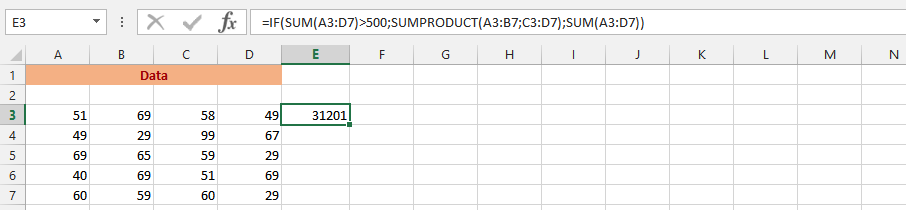=IF(SUM(A3:D7)>500,SUMPRODUCT(A3:B7,C3:D7),SUM(A3:D7))

## Template

```Further reading: